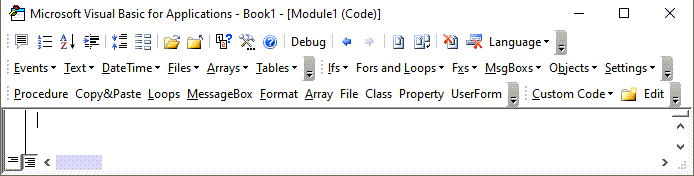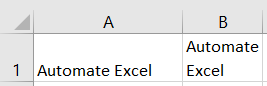# VBA Format Cells

This tutorial will demonstrate how to format cells using VBA.

## Formatting Cells

There are many formatting properties that can be set for a (range of) cells like this:

``````Sub SetCellFormat()

With Worksheets("Sheet1").Range("B5:C7")
.HorizontalAlignment = xlHAlignDistributed
.Font.FontStyle = "Italic"
.NumberFormat = "General"
.Interior.Color = RGB(128, 100, 250)
End With

End Sub
``````

Let’s see them in alphabetical order:

By setting the value of this property to True the text will be automatically indented when the text alignment in the cell is set, either horizontally or vertically, to equal distribution (see HorizontalAlignment and VerticalAlignment).

``````With Worksheets("Sheet1").Range("A1")
.Orientation = xlVertical
.VerticalAlignment = xlVAlignDistributed
End With``````

### Borders

As an example you can set a red dashed line around cell B2 on Sheet 1 like this:

``````Worksheets("Sheet1").Range("B2").BorderAround LineStyle:=xlDash, ColorIndex:=3
``````

### Font

You can adjust the cell’s font format by setting the font name, style, size, color, adding underlines and or effects (strikethrough, sub- or superscript). See here for more information about cell fonts.

Here are some examples:

``````With Range("A1:C5").Font
.Name = "Century"
.FontStyle = "Bold"
.Strikethrough = True
End With
``````

### FormulaHidden

This property returns or sets a variant value that indicates if the formula will be hidden when the worksheet is protected. For example:

`` Worksheets("Sheet1").Range("A1:B1").FormulaHidden = True``

### HorizontalAlignment

This property cell format property returns or sets a variant value that represents the horizontal alignment for the specified object. Returned or set constants can be: xlGeneral, xlCenter, xlDistributed, xlJustify, xlLeft, xlRight, xlFill,  xlCenterAcrossSelection. For example:

``````Worksheets("Sheet1").Range("D3").HorizontalAlignment = xlRight
``````

Stop searching for VBA code online. Learn more about AutoMacro - A VBA Code Builder that allows beginners to code procedures from scratch with minimal coding knowledge and with many time-saving features for all users!### IndentLevel

It returns or sets an integer value between 0 and 15 that represents the indent level for the cell or range.

``Worksheets("Sheet1").Range("A1").IndentLevel = 7``

### Interior

You can set or get returned information about the cell’s interior: its Color, ColorIndex, Pattern, PatternColor, PatternColorIndex, PatternThemeColor, PatternTintAndShade, ThemeColor, TintAndShade, like this:

``````If Not Range("A1").Interior.ThemeColor = ThemeColorLight2 Then
Range("A1").Interior.Pattern = xlPatternUp
End If
``````

### Locked

This property returns True if the cell or range is locked, False if the object can be modified when the sheet is protected, or Null if the specified range contains both locked and unlocked cells. It can be used also for locking or unlocking cells.

This example unlocks cells A1:B2 on Sheet1 so that they can be modified when the sheet is protected.

``````Worksheets("Sheet1").Range("A1:B2").Locked = False
Worksheets("Sheet1").Protect
``````

VBA Programming | Code Generator does work for you!

### MergeCells

Set this property to True if you need to merge a range. Its value gets True if a specified range contains merged cells. For example, if you need to merge the range of C5:D7, you can use this code:

``Worksheets("Sheet1").Range("C5:D7").MergeCells = True``

### NumberFormat

You can set the number format within the cell(s) to General, Number, Currency, Accounting, Date, Time, Percentage, Fraction, Scientific, Text, Special and Custom.

Here are the examples of scientific and percentage number formats:

``````Range("A1").NumberFormat = "0.00E+00"
Range("B1").NumberFormat = "0.00%"
``````

### NumberFormatLocal

This property returns or sets a variant value that represents the format code for the object as a string in the language of the user.

### Orientation

You can set (or get returned) the text orientation within the cell(s) by this property. Its value can be one of these constants: xlDownward, xlHorizontal, xlUpward, xlVertical or an integer value from –90 to 90 degrees.

``Worksheets("Sheet1").Range("A1").Orientation = -60``

### Parent

This is a read-only property that returns the parent object of a specified object.

### ShrinkToFit

This property returns or sets a variant value that indicates if text automatically shrinks to fit in the available column width.

``Worksheets("Sheet1").Range("A1").ShrinkToFit = True``

### VerticalAlignment

This property cell format property returns or sets a variant value that represents the vertical alignment for the specified object. Returned or set constants can be: xlCenter, xlDistributed, xlJustify, xlBottom, xlTop. For example:

``Worksheets("Sheet1").Range("A1").VerticalAlignment = xlTop``

### WrapText

This property returns True if text is wrapped in all cells within the specified range, False if text is not wrapped in all cells within the specified range, or Null if the specified range contains some cells that wrap text and other cells that don’t.

For example, if you have this range of cells:this code below will return Null in the Immediate Window:

``?Worksheets("Sheet1").Range("A1:B1").WrapText``